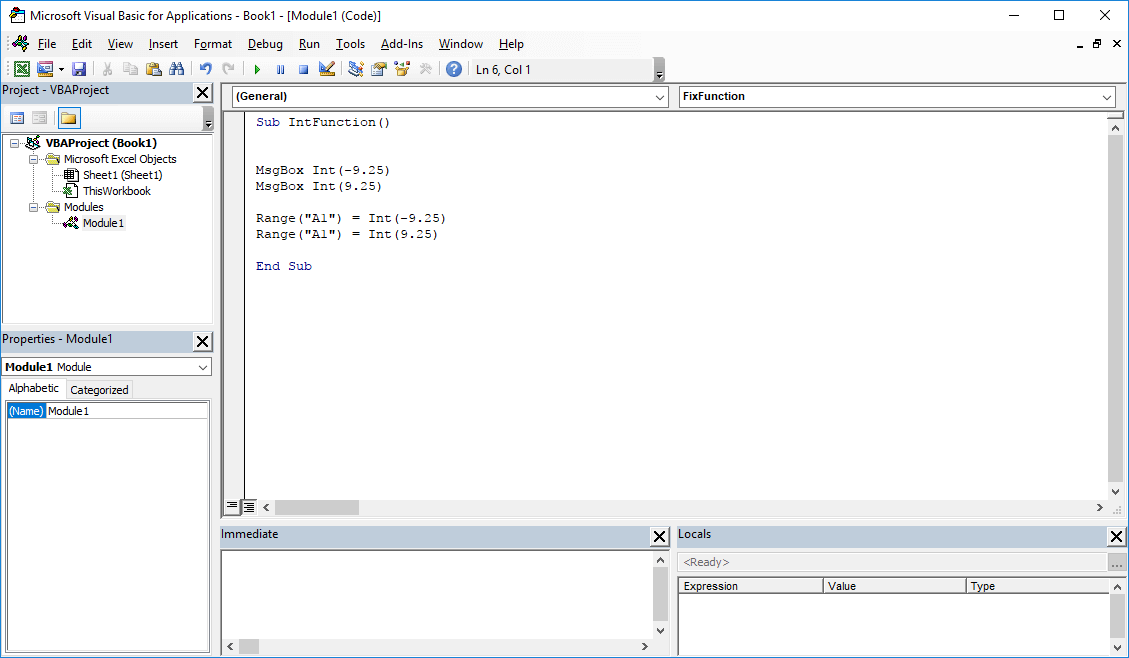# Int VBA function – How to round negative numbers down

## 1. Int VBA function – Description

The Int VBA function is used to round numbers that have decimal places to integers. The Int function, in comparision to the Round function, does not require additional arguments. The result will always be an integer value.

An important information is that, for negative numbers, the VBA Int function rounds a number down from zero. For example, the number -4.5 will be rounded to -5. If you want to round negative numbers up to zero, you should use the VBA Fix function.

## 2. VBA Int function – Syntax

```'officeinside.org
Int (Number)```

Number : The number that you want to round.

Function return : Number / integer value.

## 3. VBA Int function – Example

How to use the Int  function in VBA Excel? Examples of using the Int function in the VisualBasic Editor are listed below.

Example 1 : Example of using the Int VBA function – result in the MsgBox window.

```'officeinside.org
MsgBox Int(-9.25)
MsgBox Int(9.25)```

Example 2 : VBA Int – example of a function – result in the Excel spreadsheet.

```'officeinside.org
Range("A1") = Int(-9.25)
Range("A1") = Int(9.25)```Excel VBA functions – Int VBA function

## 4. VB Int function – Additional information

• The function does not require entering additional arguments.
• In the case of negative numbers, the function rounds down from 0.

## 5. Int VisualBasic function – Where to use?

This function can be used in: Excel 2003, Excel 2007, Excel 2010, Excel 2013, Excel 2016.

This article is a part of the list of VBA Excel functions. A list of all VBA functions can be found at this address: Excel VBA functions.